### Day 11 - Limit Problems - 09.02.14

 Bell Ringer Batavia Sports Booster AppMath Circle at WaubonseeGo over Quiz 2Find all values of c such that f is continuous on (-∞, ∞).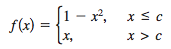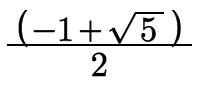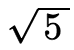both a and bnone of the aboveWhat is the domain of f(x)?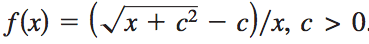x ≠ 0x ≥ -c2x > -c2both a and bnone of the aboveFind the following limit: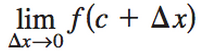given that the function is continuous throughout.0∞f(0)f(c)none of the aboveFind the following limit: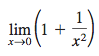∞-∞10none of the above Review Finding limits using a table Finding limits using a graph Epsilon-Delta Limit Proofs Limit Properties Dividing Out/Rationalizing Techniques Functions that agree at all but one point One-sided Limits Infinite Limits Lesson Find the values of the constants a and b such that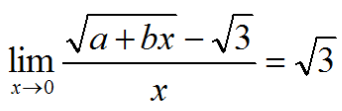Consider the function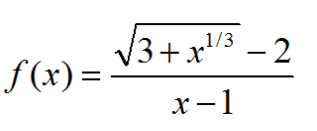Find the domain of f.Calculate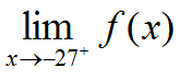Calculate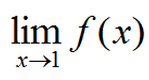Determine all values of the constant a such that the following function is continuous for all real numbers.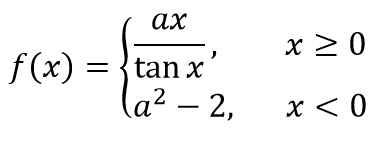Let a be a nonzero constant. Prove that if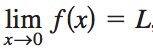, thenShow by means of an example that a must be nonzero. Exit Ticket The function f and its graph are shown below: Calculate the limit of f(x) as x gets closer to 2 from the left side. Which value is greater? the limit of f(x) as x goes to 1 f(1) Explain your answer. At what value(s) of c on the interval [0, 4] does the limit of f(x) as x goes to c not exist? Explain your answer.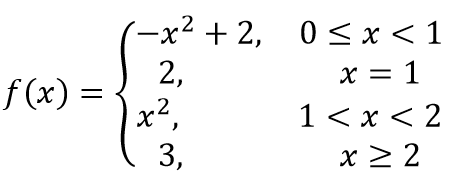Lesson Objective(s) How are the concepts in chapter 1 related and applied? Standard(s) APC.2 Define and apply the properties of limits of functions. Limits will be evaluated graphically and algebraically. Includes: ​limits of a constant ​limits of a sum, product, and quotient ​one-sided limits ​limits at infinity, infinite limits, and non-existent limits*APC.3Use limits to define continuity and determine where a function is continuous or discontinuous.Includes:​continuity in terms of limitscontinuity at a point and over a closed interval​application of the Intermediate Value Theorem and the Extreme Value Theorem​geometric understanding and interpretation of continuity and discontinuityAPC.4Investigate asymptotic and unbounded behavior in functions.Includes:describing and understanding asymptotes in terms of graphical behavior and limits involving infinity